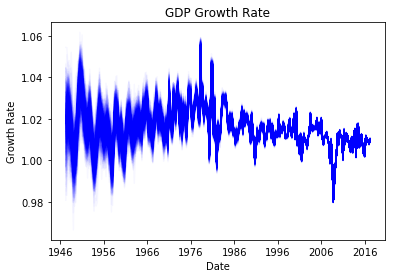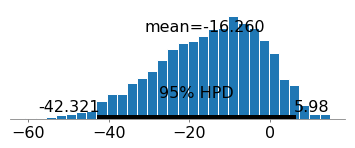### Bayesian Time Varying Coefficients in PYMC3Okay so today I want to talk about something really cool that you can do with time-series / panel data. That is, you can allow the coefficients in the model change over time. It is a really neat idea, but […]

read more →

### Bayesian Logistic Regression in Python using PYMC3In my last post I talked about bayesian linear regression. A fairly straightforward extension of bayesian linear regression is bayesian logistic regression. Actually, it is incredibly simple to do bayesian logistic regression. If you were following the last post that […]

read more →